Implementation of bidirectional ray tracing algorithm jet@inf.bme.hu Department of Control Engineering and Information TechnologyTechnical University of BudapestBudapest, HungaryAbstract: This paper examines existing bidirectional ray tracing algorithms and the bottlenecks and possible benefits compared to backward tracing methods. The results of the implementation of one algorithm is provided. The article addresses the problems in the implementation of this a system including accurate probability calculation and efficient ray generation.

Keywords: Computer graphics, ray tracing, Monte Carlo methods, bidirectional ray tracing

### 1. Introduction

The aim of computer graphics is the solution of the rendering equation [Szir95]. It causes many difficulties, that this equation contains the function to estimate on both sides. Also, the function to integrate has bad properties. The light sources in the scene are usually small, therefore most light within the scene usually comes from a small area. In many cases, the surfaces are specular, and the light is reflected into a small area as well. Monte Carlo and quasi Monte Carlo [Shri65] ray tracing methods are powerful tools to solve the integral equation, because they can provide a good estimate for the typical functions in computer graphics, where other numerical methods fail.

Monte Carlo methods were also successfully applied in the field of radiosity methods. This topic is discussed in [SzFW98b] and [SzFTCs97]. An elegant solution was proposed in [SzFW98a] that computes the radiosity of patches with an infinite number of rays using global directions. However, radiosity-based methods do provide efficient solution only if the surfaces are mostly diffuse and the light sources are large. The decomposition of large surfaces into smaller patches represents an other problem, therefore a universal solution may become relatively complex compared to the methods discussed in this paper.

The goal of the ray tracing process is to determine the amount of light arriving to the observer through the pixels of the image. To do that, Monte Carlo ray tracing algorithms generate light transport paths in the object space that carry a given amount of light from a light source to the camera, and estimate the amount of incoming light with these sample light paths. Since our computational capacity is limited, we would like to compute the image by computing as little light paths as possible. To achieve this, we need an algorithm that generates paths that carry much light (and hence contribute to the final image significantly), and that does not generate paths that carry little amount of light.

### 2. Analyzing Monte Carlo ray tracing methods

Now let us examine the existing Monte Carlo methods concentrating on these criteria. We assume, that our scene contains several objects, a pinhole camera, and several light sources. Light sources are allowed to be point light sources or area light sources.

#### 2.1 Standard Monte Carlo ray tracing

Clearly, one end of all important paths should be at the camera (we assume pinhole camera model for simplicity), and the other end must be in a point light source or on the surface of an area light source. So, an algorithm could use either the camera or the light sources as a starting point to build light paths. However, in most cases there is only one camera in the model, and there may be several light sources. Furthermore, all important paths should arrive in the single camera, in contrast to the light sources, where it is possible that the effects generated by a light source are not visible on the image at all. Because of these reasons, standard Monte Carlo path tracing methods use the single camera as a starting point for transport path generation, and trace light rays backwards.

After selecting a starting point, the algorithm chooses a random direction, and fires a ray into that direction, examining which surface is hit by the ray. When making further bounces, the further random directions are usually not chosen uniformly, but according to the Bidirectional Reflectance Distribution Function (BRDF) of the surface, i.e. the probability of choosing a direction is proportional to the BRDF. This method reduces the noise of the image since it is more likely to generate directions where the transmission is large. This idea is called importance sampling.

After a number of samples have been taken, the following equation is used to estimate of the integral: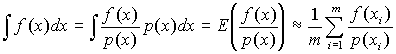(1)
The expected value of the expression is the value of the integral. The variance (noise) decreases, as the number of samples increases.

This method may produce an acceptable result, but a lot of scenes exist, where the noise level of the image generated will be far too big. To generate a path that contributes to the final image significantly, the path has to end in a light source. In case of point light sources, this would be impossible, so standard tracing techniques treat them specially, usually trying to fire a ray against all point light sources after each bounce.

If there are area light sources in the system, this tracing algorithm works fine only if the light sources are big, and the surfaces are nearly specular. In this case, the probability of randomly hitting a light source is large. But if the light sources are small and the surfaces are diffuse, the algorithm has almost no chance to hit the light source. This will introduce a lot of noise in the final image.

#### 2.2 Monte Carlo ray tracing with direct lighting component

To overcome the problem of diffuse surfaces and small light sources, the direct lighting method was proposed in [Shir91]. This method differs only in the last step, where instead of sampling the BRDF of the material, a random point is chosen on the surface of a light source, and the ray is fired against that point. This way, small light sources can be handled with clearly with much lower noise level. However, this method introduces an other problem. If we have a large light source those light is reflecting on an almost perfectly mirroring shiny surface, it has extremely low probability that any light is transmitted according to the BRDF of the surface into the randomly chosen direction, since the BRDF is significant only around the direction of perfect mirroring.

#### 2.3 Optimal combination of samples

Let us realize, that standard Monte Carlo ray tracing and the direct lighting method complete each other very well: the one performs well where the other fails and vica versa. It would be convenient, if we could generate paths with both methods, and would have an algorithm to combine samples from them.

A very elegant and efficient method was presented in [VG95]. If we have n sampling techniques, we can construct an F function based on the wi weight distribution derived from pi probabilities so that the variance of F will be provably lower than the original one: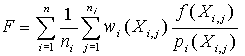(2)
where ni denotes the number of samples taken with each path generation method. The expected value of F remains unchanged: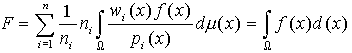(3)
If we have a number of sampling techniques, this method guarantees that the most appropriate one will suppress the others, keeping the benefits of all methods.

#### 2.4 Bidirectional ray tracing

Although standard Monte Carlo tracing and direct lighting together provide adequate sampling technique for most situations, they may still not be proper for certain types of scenes. For example, suppose, that we have a scene where the light of an area light source hits a mirror and the mirror reflects it onto a wall. In this case, the most suitable sampling technique would be to start shooting rays from the light source, bounce once, and connect this path segment to the route coming from the camera. Similar cases can be constructed for almost all kind of sampling techniques.

A more general light path generator algorithm was developed independently by Veach and Guibas [VG94, VG95] and Lafortune and Willems [LW93]. The algorithm generates light paths from both ends. One segment is built up starting from the camera while the other segment is starting from an arbitrary point on the surface of a light source. Finally, the two segments are connected with a deterministic step. The process is illustrated on Figure 2.4.Figure 2.4: The process bidirectional light path generation

From the camera, the first step is to generate a point on the lens aperture. Currently, this is a single determined point, but we leave this step because further improvements may take use of this, e.g. motion blur can be introduced if the camera points are generated on a line segment. From the light source side, the first step is to generate a point on a light emitting surface. This approach allows only area light sources. (Point light sources could be introduced easily.) The number of camera and light source steps is pre-determined.

These methods applied for all possible camera path and light source path lengths give a number of ways to generate light paths. Theoretically, the number of bounces can be very big, put in practical cases, the paths that have a length of more than 4-8 (depending on the scene), do not contribute to the picture significantly, so they can be ignored. The transmissions of paths generated by different methods can be combined by the method described in the previous chapter ([VG95]).

Implementation problems of this method is further discussed in section 4.

### 3. The TrayLib library

Although it is not strictly related to the topic of this document, a general purpose ray tracer library was developed during the development of the bidirectional ray tracer, in cooperation with others.

#### 3.1 Design goals and principles

• Allow the easy development of recursive and Monte Carlo ray tracing systems. The library should support the easy implementation of our ideas and rendering systems, including the bidirectional Monte Carlo ray tracer and a particle tracer.

• Should be portable across various computer platforms including UNIX from different vendors, DOS and Windows command prompt. This decision restricted to the number of available programming languages heavily. DOS has been a primary platform since to allow everyone to work on the project on a home computer. Most of the developments were first implemented and tested in this environment. Beyond that, current implementation runs on SGI IRIX, Linux, Sun Solaris and Windows95/NT.

• Should be object-oriented. Object-orientation's advanced features allow easier development and extension of the library.

• Should be extremely efficient in terms of computational costs. Because of that, C++ was chosen as programming language, since other object oriented languages do not allow this level of performance.

• Should support existing file formats at mesh data input, texture input and picture output as well. Currently supported formats: 3D Studio Release 4 and Materials and Geometry Format(MGF, [Ward95]) at mesh input, GIF at texture input, 24-bit PCX and TGA at picture output.

#### 3.2 Current features

The library provides a range of low level classes to create high-performance ray tracing applications: 2 and 3 dimensional vector classes, light class, buffered input and output classes, basic templated storage classes. Only Phong surface model [Phong75] is supported, but the set of models can be extended easily. Texture maps and other maps are also supported.

The library has several demo applications:

• The Trace demo is a very simple ray tracer, it completely ignores inter-reflections between surfaces, only the light incoming from non-area light sources is taken into account, and uses only one ray per pixel. Figure 3.2a shows several images rendered with this program. The office scene contains about 1300 patches and is rendered in 3 minutes on a workstation in 640 x 480 resolution.

• The Realtime demo is a performance demonstration, it uses the same rendering method as Trace, but it renders a very simple scene consisting of 4 primitives in 80 x 60 resolution at about 10 frames per second on a top PC.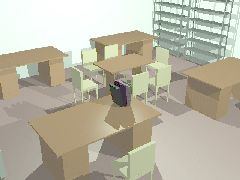Figure 3.2a: Images generated with the single-step ray tracer.
• The Puremc demo performs standard Monte Carlo ray tracing as described in section 2.1. Figure 3.2b shows the office scene rendered with Puremc. The scene was rendered at 20 samples per pixel under the same circumstances as with Trace. The total rendering time in this case was 60 minutes.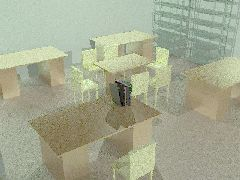Figure 3.2b: Image generated with the standard Monte Carlo ray tracer.

#### 3.3 Monte Carlo functionality

The core of Monte Carlo functionality is implemented in the Material class. All subclasses deriving from this class should have the following virtual methods:

Light getF(const Vector &in, const Vector &out, const Surface &s):
Returns the actual value of BRDF for the given incoming and outgoing directions (class Surface contains the surface normal).
void generatePath(const Vector &in, Vector &out, const Surface &s):
Chooses a random outgoing direction from the surface. The probability of directions should be proportional to the value of f, if possible.
double getP(const Vector &in, const Vector &out, const Surface &s);
Returns the value of the probability density function of choosing out as an outgoing path in the previous method.

Existing Monte Carlo ray tracer implementations generally do not calculate the value of f and p separately, since only f/p is required in standard ray tracing methods (as seen in (2) ) that can be calculated at lower cost than determining f and p independently. However, the optimal combination of samples (section 2.3) from several sampling techniques requires the explicit value of pi to calculate the wi weights.

### 4. The implementation of the bidirectional ray tracer

This section introduces the problems of the implementation of the bidirectional ray tracing using the algorithm described in [VG95] and the TrayLib library. Because of realism, only area light sources are allowed in the system.

To determine the intensity value of a pixel in the image, a number of samples light paths are taken that go through the pixel. The integral is estimated with an improved version of the formula in (1). To calculate the estimated value, the evaluation of f(x) and p(x) is required for all generated light paths.

#### 4.1 Determining the value of f(x)

The value of f(x) represents the amount of light intensity arriving through a through that light path. The Iout intensity leaving a light emitting surface can be derived from the properties of the surface (usually constant in all directions within one patch). As the ray bounces at a number of patches, the intensity it transmits changes according to (4):
 Iout=Iin·BRDF(L,V)·cos(Theta) (4)
where L and V are the incoming and outgoing light directions and Theta is the angle between L and the surface normal. The value of the BRDF function can be easily determined using the methods of the Material class.

#### 4.2 Determining p(x)

The value of p(x) is tells the value of probability density function for the whole path, hence it is more difficult to calculate. p(x) can be interpreted, as a probability distribution of paths on the computer screen, that has to be determined for all paths.

First, let us examine the case of standard Monte Carlo ray tracing, where rays are always traced backwards. In this case, p(x) can be calculated as a product of the values of the local probability functions: p0 is the value of probability density function of generating the first ray from the camera, while pi are the probability density functions of generating bounces respectively.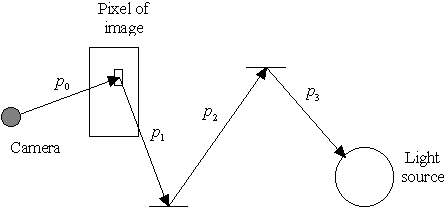Figure 4.2a: Calculating p(x) in the standard Monte Carlo case

When tracing the path of light from the light sources, we need to calculate the same function. However, when tracing the path of light from the light sources, we do not generate the path according to these probabilities, so the probability density has to be transformed into our system. The transformation for the first step - when a random point is chosen on a light emitting surface - is shown on Figure 4.2b: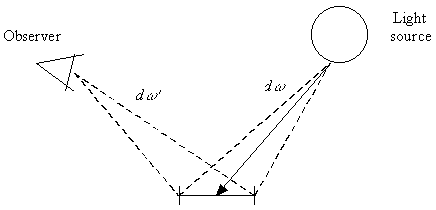Figure 4.2b: Transforming the probability of direct lighting component

The transformed probabilities can be calculated with the following formulae: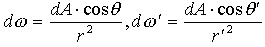(5)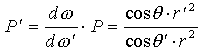(6)
The transformation of further bounces from the light source goes similarly. This process has to be repeated for all steps coming from the light source before the values of local probability density functions can be multiplied.

#### 4.3 The results

The process shown is capable of producing good images. In the images shown, the length of light paths is limited to 4 or 5 steps, that is equivalent of selecting the camera location, a point on a light emitting surface and two bounces between them. Practical results show that taking longer paths into account would not contribute to the images significantly.

For efficiency, light paths are not generated fully independently of each other: shorter paths are generated from longer paths by leaving steps out of them. This method does not introduce any visible artifacts to the image generated but reduces computational costs. The scenes shown below contain 12-17 geometric primitives while the computation time was between 60 and 90 minutes on a workstation for one image, with 200-300 samples per pixel in 640 x 480 resolution.

Figure 4.3a shows a Cornell-box like scene with an almost perfectly mirroring big metal ball and a smaller, purple but very shiny ball. Shadows are soft because of the large light source. Note that diffuse inter-reflection is also taken into account, the effects can be observed especially on the ceiling, where the colors of neighboring walls are reflected next to the light source.

Figure 4.3b shows a similar scene. The light source is much smaller but the intensity is bigger, so that the total flux emitted is the same as in the previous case. This shows that the method is equally suitable for small and large light sources. The small light ball to the right is an imperfect mirror. Note that not only the area light source, but the reflection of the walls are rendered in high quality.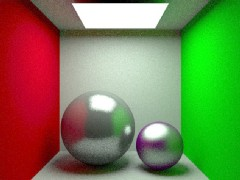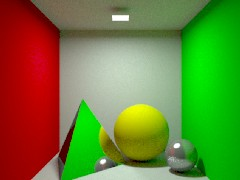Figure 4.3a and 4.3b: Images generated with the bidirectional path tracer
Figure 4.3c shows an interesting scene that is believed to be a hard case for non-bidirectional ray tracers: the almost perfectly mirroring pyramid reflects the light of the light source to the wall. This reflection would be almost impossible to trace with non-bidirectional methods in reasonable quality, because it is very unlikely that a randomly selected direction from the floor will hit the small light source. On the other hand, direct lighting would be also unsuitable, because it can be applied only in the last step of the tracing.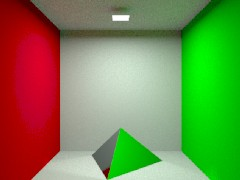Figure 4.3c: Light mirrored onto the floor

### 5. Conclusions and future work

We provided an overview of standard and bidirectional Monte Carlo ray tracing methods. We showed that bidirectional tracing is more efficient in many situations where standard Monte Carlo methods do not produce accurate results. We provided an implementation of a bidirectional ray tracer, and showed that this method works in practice.

Future work should should concentrate primarily on further improving the algorithm, examining current more advanced methods such as in [VG97]. Also, the implementation of both TrayLib and the bidirectional renderer can be improved in many fields.

### 6. Credits

First of all, I would like to say thanks to László Szirmay-Kalos, my supervisor who helped me in the development of ideas and algorithms. I would like to give credits to Dénes Bezzeg and István Márk Rázsó, who took part in the implementation of TrayLib. The research work was supported by the Hungarian National Scientific Research Fund (OTKA), project ref. No. 015884; and the Austrian Academic Exchange Service (ÖAD), project ref. No. 32öu9 and 29p4.

### 7. Bibliography

 [LW93] Lafortune, E. and Willems, Y.D.: Bi-directional Path-Tracing Compugraphics '93 [Phong75] Phong, Bui Thong: Illumination for Computer Generated Images Communications of the ACM journal, Volume 18, 1975 [Shir91] Peter Shirley and Wang : Direct Lighting Calculation by Monte Carlo Integration Proceedings of the 2nd Eurographics rendering Workshop, Barcelona, 1991 [Shri65] Shrider, J. (editor): The Monte-Carlo Method Pergamon Press, Oxford, 1966 [Szir95] László Szirmay-Kalos: Theory of three-dimensional computer graphics Akadémiai Kiadó, Budapest, 1995 [SzFW98a] László Szirmay-Kalos, Tibor Fóris, Werner Purgathofer: Quasi-Monte Carlo Global Light Tracing with Infinite Number of rays Winter School of Computer Graphics, 1998 [SzFW98b] László Szirmay-Kalos, Tibor Fóris, Werner Purgathofer: Non-diffuse, Random-walk Radiosity Algorithm with Linear Basis Functions Journal of Machine Graphics and Vision, 1998 May [SzFTCs97] László Szirmay-Kalos, Tibor Fóris, László Neumann, Balázs Csébfalvi: An Analysis of quasi-Monte Carlo Integration Applied to the Transillumination Radiosity Methods Computer Graphics Forum, Vol 16, No 3, 1997 [VG94] Eric Veach and Leonidas J. Guibas: Bidirectional Estimators for Light Transport Proceedings of Fifth Eurographics Workshop on Rendering, 1994 [VG95] Eric Veach and Leonidas J. Guibas: Optimally Combining Sampling Techniques for Monte Carlo Rendering Proceedings of SIGGRAPH 95 [VG97] Eric Veach and Leonidas J. Guibas: Metropolis Light Transport Proceedings of SIGGRAPH 97 [Ward95] Greg Ward: The Materials and Geometry Format Lawrence Berkeley Laboratory, 1995